New update is available. Click here to update.
Last Updated: Jun 30, 2023
Easy

# Operators in Ruby

## Introduction

Hello and welcome, readers! We hope you are doing well.

Today, we will discuss operators in Ruby. An operator is one of the basic building blocks of a programming language that enables us to perform different operations in a programming language. Ruby provides various operators such as arithmetic, logical, comparison, bitwise, etc. We will discuss each of them one by one. So follow the article till the end.

So, without further ado, let’s jump into the article.

## Ruby Operators

An operator is nothing but a symbol used to represent an operation that can be performed on single or multiple operands. There are different operators present in Ruby. In the subsequent sections, we will give an insight into different types of operators and their implementation in the Ruby programming language.

### Arithmetic Operators

Arithmetic operators are used for arithmetic or mathematical operations.

Below are the different arithmetic operators available in Ruby: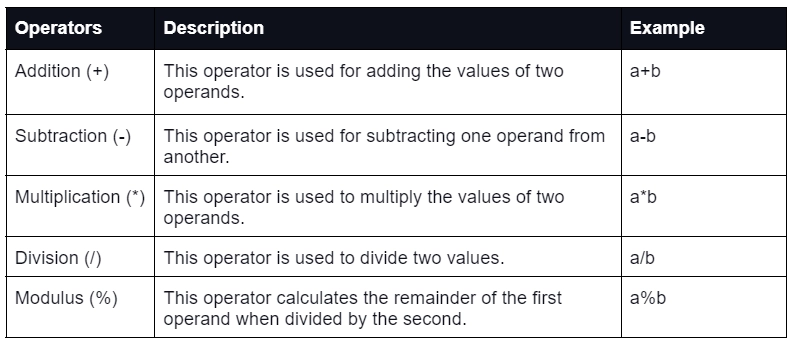In the above section, we discussed the arithmetic operators in Ruby programming language and their descriptions. Now, look at the code below to implement some of the operators mentioned above.

Example:

``````#program for different operators
a = 6
b = 3
puts "a = #{a}"
puts "b = #{b}"
#arithmetic operators
puts "--------------------"
puts "Arithmetic operators:"
puts "Subtraction: #{a-b}"
puts "Multiplication: #{a*b}"
puts "Division: #{a/b}"
puts "Modulus: #{a%b}"
puts "Exponent: #{a**b}"``````

Output:

``````a = 6
b = 3
--------------------
Arithmetic operators:
Subtraction: 3
Multiplication: 18
Division: 2
Modulus: 0
Exponent: 216``````

### Comparison Operators

Comparison operators are also called relational operators. It is used to compare two values. Let's see the comparison operators available in Ruby.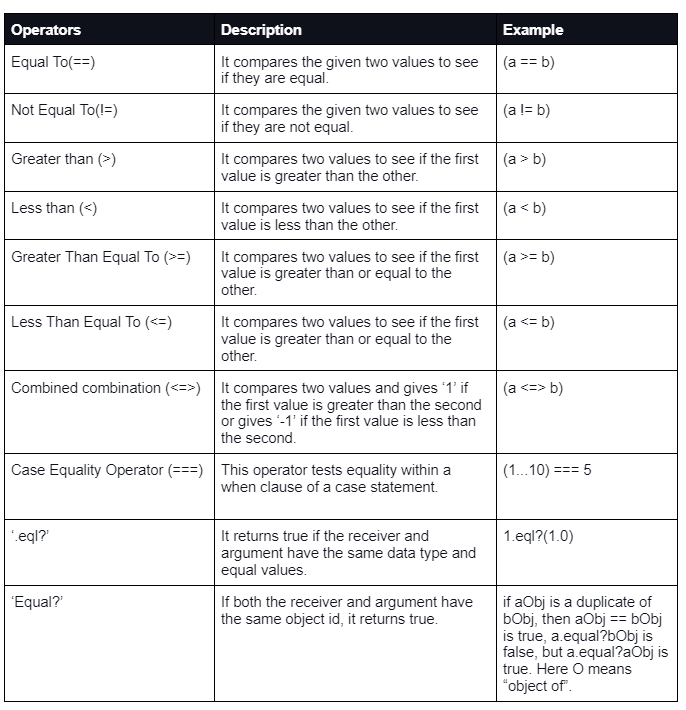In the above section, we discussed the comparison operators in the Ruby programming language and their descriptions. Now, let's look at the code below to implement some of the operators mentioned above.

Example:

``````#program for different operators
a = 6
b = 3
puts "a = #{a}"
puts "b = #{b}" 6 #comparison operators 7 puts "----
puts "Comparison operators:"
puts "a == b: #{a == b}"
puts "a != b: #{a != b}"``````

Output:

``````a = 6
b = 3
--------------------
Comparison operators:
a == b: false
a != b: true``````

### Logical Operators

These operators are used for combining two or more conditions. The following are the logical operators available in Ruby.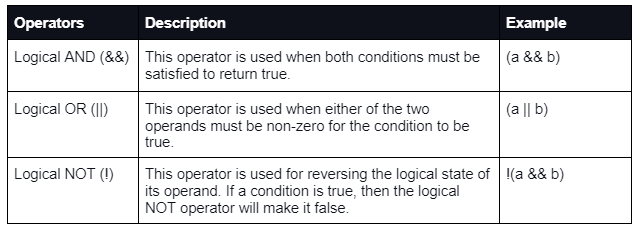In the above section, we discussed the logical operators in Ruby programming language and their descriptions. Now, let’s look at the code below to practically implement some of the operators mentioned above.

Example:

``````#program for different operators
a = 6
b = 3
puts "a = #{a}"
puts "b = #{b}"
#logical operators
puts "-----------------"
puts "Logical operators:"
puts "a >= b: #{a >= b}"
puts "a <= b: #{a <= b}"``````

Output:

``````a = 6
b = 3
-----------------
Logical operators:
a >= b: true
a <= b: false``````

### Assignment Operators

It is used for assigning a value to a variable. The operand on the left of the assignment operator is a variable, and the operand on the right is a value. The right value must be the same data type as the variable on the left. Otherwise, the compiler will issue an error.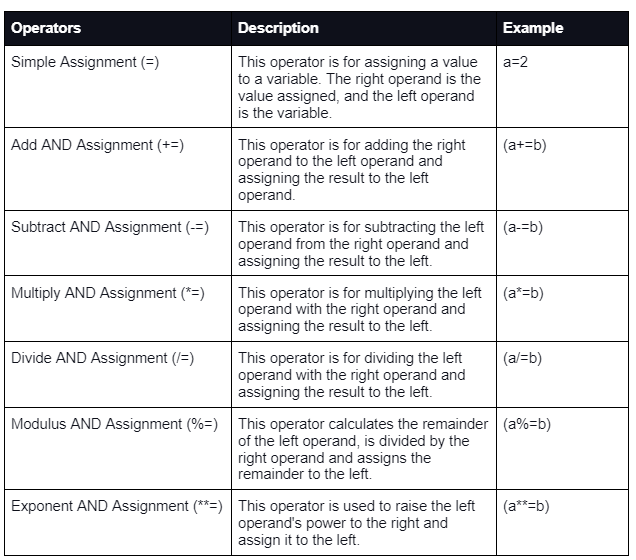In the above section, we discussed the assignment operators in Ruby programming language and their descriptions. Now, let's look at the code below to implement some of the operators mentioned above practically.

Example:

``````#program for different operators
a = 6
b = 3
puts "a = #{a}"
puts "b = #{b}"
#assignment operators
puts "-----------------"
puts "Assignment operators:"
a += b
a -= b
puts "Subtraction: #{a}"``````

Output:

``````a = 6
b = 3
--------------------
Assignment operators:
Subtraction: 6``````

### Bitwise Operators

These operators work at the bitwise level or are used to perform bitwise operations. The following are the bitwise operators available in the Ruby programming language.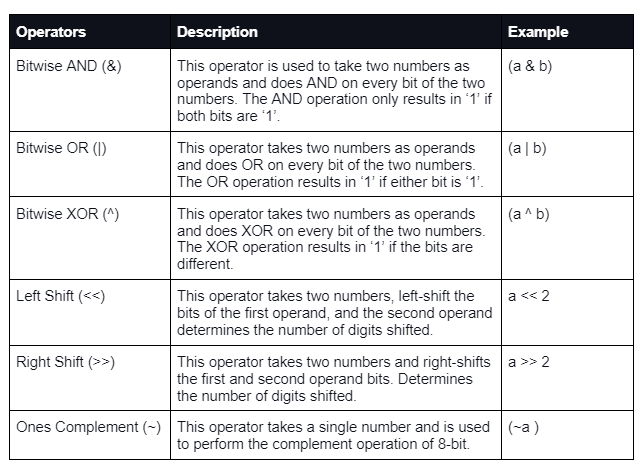### Ternary Operators

This operator first evaluates an expression to a true or false value and then executes one of two given statements depending on the evaluation result. It is called the ternary operator because of having three operators.

In the above section, we discussed the ternary operators in the Ruby programming language and their descriptions. Now, let's look at the code below to implement some of the operators mentioned above.

Example:

``````#program for different operators
a = 6
b = 3 puts "a = #{a}"
puts "b = #{b}"
#ternary operator
puts "-----------------"
puts "Ternary operator:"
puts "Pass Marks: 40"
puts "Enter marks obtained :" marks = gets.chomp.to_i
puts "You have #{marks >= 40 ? "passed" : "failed"} the exam"``````

Output:

``````a = 6
b = 3
-----------------
Ternary operator:
Pass Marks: 40
Enter marks obtained:``````

### Range Operators

The range operators create a particular sequence range of specific elements. There are two range operators in Ruby.

### Defined? Operators

The defined? operator is a special operator used to check whether the passed expression is defined or not.

### Dot "." and Double Colon "::" Operators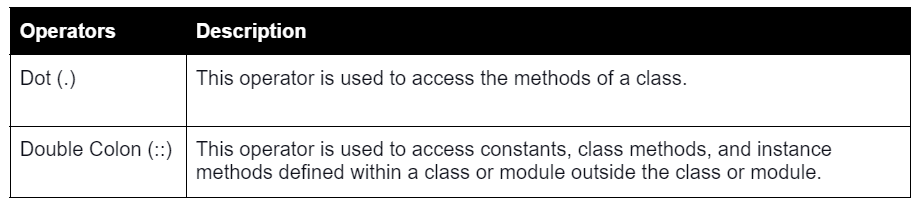### Is Ruby a compiled language?

Ruby is similar to Java in that it is a compiled language. Ruby is compiled into a collection of bytecode instructions processed by a virtual machine rather than native machine code.

### Why is Ruby an object-oriented programming language?

A Ruby module is an essential component of Ruby's programming language. It's a key object-oriented language element and indirectly allows multiple inheritances. A module can contain classes, methods, constants, and other modules.

### Is Ruby commonly used in web development?

Ruby is a well-liked, versatile programming language that is in high demand. It has several applications, including scripting, web development, DevOps, data processing, static site generation, etc. So, we can commonly use Ruby in web programming.

### Why is Ruby so famous?

This language is designed to be productive and fun for programmers. Developers like to use Ruby because it is high-level and has a simple syntax. It requires less code and focuses mainly on finding a solution to the problem.

## Conclusion

This article extensively discussed the operators we use in the Ruby programming language and their implementation. We can implement these operators using the compiler Rubinius.

We hope this blog has helped you gain insight into the Ruby programming language and its different operators. You can also check out our articles on Ruby vs PythonRuby on rails for your next web development projectCareer Opportunities after mastering Ruby on RailsRuby and Ruby on Rails: How do they differ?. Do upvote our blog to help other ninjas grow.

Head over to our practice platform Coding Ninjas Studio to practice top problems, attempt mock tests, read interview experiences, interview bundle, follow guided paths for placement preparations and much more!